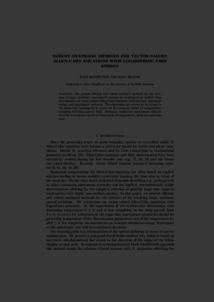Repository: Freie Universität Berlin, Math Department

# Robust multigrid methods for vector-valued Allen-Cahn equations with logarithmic free energy

Kornhuber, R. and Krause, R. (2006) Robust multigrid methods for vector-valued Allen-Cahn equations with logarithmic free energy. Computing and Visualization in Science, 9 (2). pp. 103-116. ISSN 1432-9360Preview

2MB

Official URL: http://doi.org/10.1007/s00791-006-0020-2

## Abstract

We present efficient and robust multigrid methods for the solution of large, nonlinear, non-smooth systems as resulting from implicit time discretization of vector-valued Allen-Cahn equations with isotropic interfacial energy and logarithmic potential. The algorithms are shown to be robust in the sense that convergence is preserved for arbitrary values of temperature, including the deep quench limit. Numerical experiments indicate that the convergence speed as well is independent of temperature.

Item Type: Article Mathematical and Computer Sciences > Mathematics > Numerical Analysis Department of Mathematics and Computer Science > Institute of Mathematics 1826 Ekaterina Engel 06 Mar 2016 12:32 03 Mar 2017 14:42

Repository Staff Only: item control page